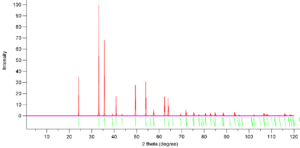# XRD Pattern Simulator – C++ Program

Recently, for my nanoscience lab, I was required to work with diffractometers, and understand the whole process.

As many of you might already know, an XRD pattern acts like a ‘finger-print’ for solid crystals, and thus is used for identification or confirmation of the sample prepared.

So let’s say you wanted to see if the sample you prepared was the one you wanted or not, then you would search the ICDD database for it’s PDF(Powder Diffraction File) and then compare this with the XRD pattern you obtained from your sample.

However, this year I was also involved in a project on DFT(Density Functional Theory), where I used a particularly amazing software called VESTA. VESTA allows you to simulate a powder XRD pattern for your crystal structure. The simulated patterns were indeed very accurate.So this could indeed be used to confirm your sample’s crystal structure, if you don’t have access to it’s particular ICDD-PDF or if the sample you’ve prepared has never been studied before.

Now since, I was studying XRD myself, I wanted to know how this had been achieved.

Even more so, when I remembered the Richard Feynman quote-

What I can’t create, I don’t understand.

So I decided to create an XRD simulator myself.

The idea is, the program would ask the user about the lattice type and the lattice parameters. And then go on to show the peak positions their miller indices, intensities, etc.

I have managed to achieve the first two things for a limited number of lattice types, however, it’s still not how good I would want it to be.

Here are the things that the program does:
1. Asks the user to enter the lattice type and parameters.
2. Based on those returns the peak positions and their corresponding miller indices.
3. User can also see the multiplicity of each peak by analysing the output.
4. Works for isoelectronic atoms and only a limited lattice types.

The code is really incomplete and lacks a lot of things, as it was created in a lot of hurry.
I just wanted to see if what I was thinking was correct or not.
This page would be updated with better versions of the code. I would really appreciate it, if someone could contribute to the code.

The code gave good results for : Si(DFCC), Ge(DFCC), KCl(FCC), Fe(BCC), Cu(FCC), and other isoelectronic species.

### CODE:

```/*************************************************************
XRD Pattern Simulator - (c) 2017 Manas Sharma
The program currently gives suitable results for single atom/isoelectronic atoms,
since the structure factor calcualtions have not yet been implemented.

One may still get an idea for mixed atom structures, but only at their own risk.

The author is not responsible for any incorrect results.

The program supports only a limited number of bravais lattices, namely:
Simple Cubic(1), FCC(2), BCC(3), Tetragonal(4), Orthorhombic(5), Hexagonal(6), Diamond FCC(7)
***************************************************************/
#include<iostream>
#include<math.h>
using namespace std;
bool isEven(int n){
if(n%2==0){
return true;
}
else
return false;
}
int main(){
//int n=25;
double lambda=1.54059;
//double theta[n];
//double d[n];
double thet,di;
double dmin=lambda/2;
int latt;
int h,k,l;
double a,b,c;
double alpha, beta, gamma;
cout<<"Enter the lattice type:\n";
cin>>latt;
switch(latt){
case 1: //Simple Cubic
cout<<"Enter the lattice parameter a:\n";
cin>>a;
b=a;
c=a;
break;
case 2: //FCC
cout<<"Enter the lattice parameter a:\n";
cin>>a;
b=a;
c=a;
break;
case 3: //BCC
cout<<"Enter the lattice parameter a:\n";
cin>>a;
b=a;
c=a;
break;
case 4: //Tetragonal
cout<<"Enter the lattice parameter a:\n";
cin>>a;
b=a;
cout<<"Enter the lattice parameter c:\n";
cin>>c;
break;
case 5: //Orthorhombic
cout<<"Enter the lattice parameter a:\n";
cin>>a;
cout<<"Enter the lattice parameter b:\n";
cin>>b;
cout<<"Enter the lattice parameter c:\n";
cin>>c;
break;
case 6: //Hexagonal
cout<<"Enter the lattice parameter a:\n";
cin>>a;
b=a;
cout<<"Enter the lattice parameter c:\n";
cin>>c;
break;
case 7: //Diamond FCC
cout<<"Enter the lattice parameter a:\n";
cin>>a;
b=a;
c=a;
break;
default:
cout<<"Enter a valid input";
return 0;
}

//cin>>alpha;
//cin>>beta;
//cin>>gamma;

for(thet=1;thet<=90;thet=thet+0.001){
di=lambda/(2*sin(thet*M_PI/180/2));
//cout<<di;
//cout<<a<<" "<<b<<" "<<c;
for(h=-a/dmin;h<=a/dmin;h++){
for(k=-b/dmin;k<=b/dmin;k++){
for(l=-c/dmin;l<=c/dmin;l++){
switch(latt){
case 1:
if(fabs(1/(di*di)-(double)(h*h+k*k+l*l)/(a*a))<=0.00001){
cout<<thet<<" ";
cout<<h<<" "<<k<<" "<<l<<"\n";
}
break;
case 2:
if(fabs(1/(di*di)-(double)(h*h+k*k+l*l)/(a*a))<=0.00001){
if(isEven(h)&&isEven(k)&&isEven(l)){
cout<<thet<<" ";
cout<<h<<" "<<k<<" "<<l<<"\n";
}
else if(!isEven(h)&&!isEven(k)&&!isEven(l)){
cout<<thet<<" ";
cout<<h<<" "<<k<<" "<<l<<"\n";
}
}
break;
case 3:
if(fabs(1/(di*di)-(double)(h*h+k*k+l*l)/(a*a))<=0.00001){
if(isEven(h+k+l)){
cout<<thet<<" ";
cout<<h<<" "<<k<<" "<<l<<"\n";
}
}
break;
case 4:
if(fabs(1/di/di-(h*h+k*k)/(a*a)-l*l/c/c)<=0.00001){
cout<<thet<<" ";
cout<<h<<" "<<k<<" "<<l<<"\n";
}
break;
case 5:
if(fabs(1/di/di-(h*h)/(a*a)-(k*k)/(b*b)-l*l/c/c)<=0.00001){
cout<<thet<<" ";
cout<<h<<" "<<k<<" "<<l<<"\n";
}
break;
case 6:
if(fabs(1/di/di-4*(h*h+k*k+h*k)/(3*a*a)-l*l/c/c)<=0.000005){
cout<<thet<<" ";
cout<<h<<" "<<k<<" "<<l<<"\n";

}
break;
case 7:
if(fabs(1/(di*di)-(double)(h*h+k*k+l*l)/(a*a))<=0.00001){
if((isEven(h)&&isEven(k)&&isEven(l))&&(abs((h+k+l)%4)<=0.01)){
cout<<thet<<" ";
cout<<h<<" "<<k<<" "<<l<<"\n";
}
else if(!isEven(h)&&!isEven(k)&&!isEven(l)){
cout<<thet<<" ";
cout<<h<<" "<<k<<" "<<l<<"\n";
}
}
break;
default:
break;
}
}
}
}
}

}
```[wpedon id="7041" align="center"]